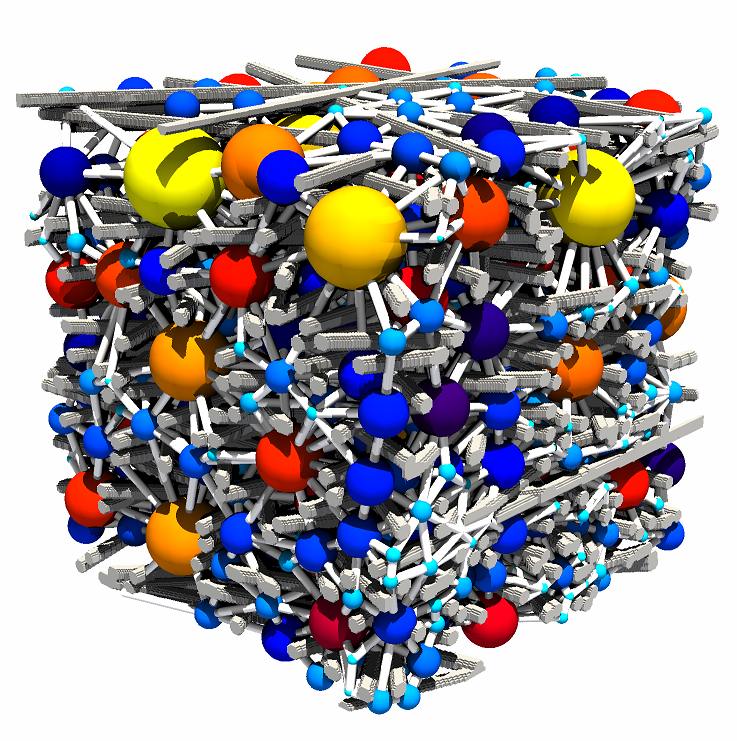The SNOW algorithm, published in Physical Review E, uses a marker-based watershed segmentation algorithm to partition an image into regions belonging to each pore. The main contribution of the SNOW algorithm is to find a suitable set of initial markers in the image so that the watershed is not over-segmented. SNOW is an acronym for Sub-Network of an Over-segmented Watershed. PoreSpy includes a predefined function called snow that applies all the steps automatically, but this example will illustrate the individual steps explicitly on a 3D image.

Start by importing the necessary packages:

:

import imageio
import numpy as np
import porespy as ps
import openpnm as op
import scipy.ndimage as spim
import matplotlib.pyplot as plt
from porespy.filters import find_peaks, trim_saddle_points, trim_nearby_peaks
from porespy.tools import randomize_colors
from skimage.segmentation import watershed

ps.settings.tqdm['disable'] = True
ps.visualization.set_mpl_style()
np.random.seed(10)


## Generate artificial image¶

One of the main aims when developing the SNOW algorithm was to extract networks from images other than sandstone, which is the main material studied by geoscientists. For this demonstration we’ll use a high porosity (>85%) image of fibrous media.

:

im = ps.generators.cylinders(shape=[300, 300, 300], r=5, ncylinders=200)
fig, ax = plt.subplots()
ax.imshow(ps.visualization.show_planes(im), cmap=plt.cm.bone);## Step-by-step application of the SNOW algorithm¶

Fist let’s find all the peaks of the distance transform which are theoretically suppose to lie at the center of each pore region. In reality this process finds too many peaks, but we’ll deal with this later.

:

sigma = 0.4
dt = spim.distance_transform_edt(input=im)
dt = spim.gaussian_filter(input=dt, sigma=sigma)
peaks = find_peaks(dt=dt)


The gaussian_filter is applied to the distance transform before finding the peaks, as this really reduces the number of spurious peaks be blurring the image slightly. The next few steps use custom made functions to help filter out remaining spurious peaks. The values of sigma and r are both adjustable but the defaults are usually acceptable.

:

print('Initial number of peaks: ', spim.label(peaks))
print('Peaks after trimming saddle points: ', spim.label(peaks))
peaks = trim_nearby_peaks(peaks=peaks, dt=dt)
peaks, N = spim.label(peaks)
print('Peaks after trimming nearby peaks: ', N)

Initial number of peaks:  743
Peaks after trimming saddle points:  732
Peaks after trimming nearby peaks:  532


The final image processing step is to apply the marker-based watershed function that is available in scikit-image to partition the image into pores. This function is wrapped by the PoreSpy function partition_pore_space. watershed can be called directly, but remember to invert the distance transform so that peaks become valleys (just multiply by -1). This step is the slowest part of the process by far, but could be sped up if a faster implementation of watershed is used. The 300**3 image used in this example will take about 1 minute to complete.

:

regions = watershed(image=-dt, markers=peaks, mask=dt > 0)
regions = randomize_colors(regions)


This should produce an image with each voxel labelled according to which pore it belongs. The patches seem to be a bit odd looking but this is just an artifact of considering a 2D slice through a 3D image.

:

fig, ax = plt.subplots()
ax.imshow((regions*im)[:, :, 100], cmap=plt.cm.nipy_spectral);Finally, this partitioned image can be passed to the network extraction function which analyzes the image and returns a Python dict containing the numerical properties of the network.

:

net = ps.networks.regions_to_network(regions*im, voxel_size=1)


This network can be opened in OpenPNM with ease, and then exported as a VTK file for viewing in ParaView.

:

prj = op.io.PoreSpy.import_data(net)


You can inspect the project and see that the network and geometry objects are present:

:

print(prj)

――――――――――――――――――――――――――――――――――――――――――――――――――――――――――――――――――――――――――――――
Object Name     Object ID
――――――――――――――――――――――――――――――――――――――――――――――――――――――――――――――――――――――――――――――
net_01          <openpnm.network.GenericNetwork object at 0x1efac42b180>
geo_01          <openpnm.geometry.Imported object at 0x1efac452950>
――――――――――――――――――――――――――――――――――――――――――――――――――――――――――――――――――――――――――――――


Overlaying the network and the image is requires using paraview since the image is in 3D. The is one gotcha due to the differences in how paraview and numpy number axes: it is necessary to rotate the image using ps.tools.align_image_with_openpnm:

:

im = ps.tools.align_image_with_openpnm(im)
imageio.volsave('image.tif', np.array(im, dtype=np.int8))

:

op.io.VTK.export_data(prj)


Finally, you can open both the tif image and the vtk file containing the network in paraview. After some fine tuning, you should be able to see something like the following: